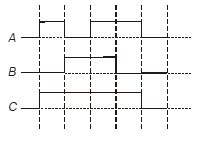Logic gates
Question

# The waveform of input A and B and the output C is given below. The logic circuit gate isEasy
Solution

## A = 1                               B = 0                            C = 1                            1 + 0 = 1          A = 0                               B = 1                            C = 1                            0 + 1 = 1          A = 1                               B = 1                            C = 1                            1 + 1 = 1OR gate.

Get Instant Solutions